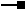# IRasterStatistics Interface

## Members

Name DescriptionIgnoredValues Array of doubles indicating the pixel values not included in the statistics calculation.IsValid Indicates if statistics are fresh.Maximum Approximate largest value.Mean Approximate average value.Median Divides pixel population approximately in halves.Minimum Approximate smallest value.Mode Approximate most popular pixel value.RasterBand The RasterBand.Recalculate Recalculate statistics based upon current skip factors and ignored values.SkipFactorX Number of horizontal pixels between samples for purposes of calculating statistics.SkipFactorY Number of vertical pixels between samples for purposes of calculating statistics.StandardDeviation Measures spread of pixel values about the mean.

### IRasterStatistics.IgnoredValues Property

Array of doubles indicating the pixel values not included in the statistics calculation.

``````Public Property IgnoredValues As Object
``````
``````public object IgnoredValues {get; set;}
``````

#### Remarks

The ignore value is ignored if calculating statistics on a grid.

### IRasterStatistics.IsValid Property

Indicates if statistics are fresh.

``````Public Property IsValid As Boolean
``````
``````public bool IsValid {get; set;}
``````

### IRasterStatistics.Maximum Property

Approximate largest value.

``````Public Property Maximum As Double
``````
``````public double Maximum {get; set;}
``````

### IRasterStatistics.Mean Property

Approximate average value.

``````Public Property Mean As Double
``````
``````public double Mean {get; set;}
``````

### IRasterStatistics.Median Property

Divides pixel population approximately in halves.

``````Public ReadOnly Property Median As Double
``````
``````public double Median {get;}
``````

### IRasterStatistics.Minimum Property

Approximate smallest value.

``````Public Property Minimum As Double
``````
``````public double Minimum {get; set;}
``````

### IRasterStatistics.Mode Property

Approximate most popular pixel value.

``````Public ReadOnly Property Mode As Double
``````
``````public double Mode {get;}
``````

### IRasterStatistics.RasterBand Property

The RasterBand.

``````Public WriteOnly Property RasterBand
``````
``````public void RasterBand {set;}
``````

### IRasterStatistics.Recalculate Method

Recalculate statistics based upon current skip factors and ignored values.

``````Public Sub Recalculate ( _
)
``````
``````public void Recalculate (
);
``````

### IRasterStatistics.SkipFactorX Property

Number of horizontal pixels between samples for purposes of calculating statistics.

``````Public Property SkipFactorX As Integer
``````
``````public int SkipFactorX {get; set;}
``````

#### Remarks

The skip factor is ignored, always use 1, if calculating statistics on a grid.

### IRasterStatistics.SkipFactorY Property

Number of vertical pixels between samples for purposes of calculating statistics.

``````Public Property SkipFactorY As Integer
``````
``````public int SkipFactorY {get; set;}
``````

#### Remarks

The skip factor is ignored, always use 1, if calculating statistics on a grid.

### IRasterStatistics.StandardDeviation Property

``````Public Property StandardDeviation As Double
``````
``````public double StandardDeviation {get; set;}
``````

## Classes that implement IRasterStatistics

Classes Description
RasterStatistics A raster statistics class.

## Remarks

The RasterStatistics class is not cocreatable and must be retrieved from a RasterBandobject. The statistics only provide information about a single band and are used when displaying the raster. The statistics may be modified or recalculated using this object.

Statistics can be calculated by setting a skip factor to avoid calculating on a full raster. Although the statitics calculated this way is not true statstics of the raster, it can reduce the calculating time significantly for large images without noticeable decreasing of the display quality.